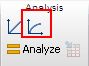## Please enable JavaScript to view this site.

 How to fit a model with nonlinear regression

## Step 1. Enter data

Create an XY table, and enter data. If you have replicate Y values at each X value, format the table for entry of replicates.

From an XY table or graph, click the shortcut button to fit a model with nonlinear regression. Or click Analyze and select from the analyze dialog.## Step 2. Choose a model

Nonlinear regression fits a model to your data. You must, therefore, choose a model or enter a new model.

## Step 3. Choose (or review) initial values

Nonlinear regression is an iterative procedure. The program must start with estimated values for each variable that are in the right "ball park" - say within a factor of five of the actual value. It then adjusts these initial values to improve the fit.  See How nonlinear regression works.

GraphPad Prism provides initial values automatically if you use a built-in equation. If you enter your own equation, you can also enter rules for initial values. For example the initial value of one parameter may be twice the maximum Y value in the data, while the initial value of another parameter may equal the average of the highest and lowest X values. Once you define these rules, Prism will compute appropriate initial values based on the range of your data.

The Initial Values tab of the Nonlinear regression dialog lets you review, and override, the computed initial values.

You'll find it easy to estimate initial values if you have looked at a graph of the data, understand the model, and understand the meaning of all the parameters in the equation. Remember that you just need an estimate. It doesn't have to be very accurate. If you are having problems estimating initial values, set aside your data and simulate curves using the model. Change the variables one at a time, and see how they influence the shape of the curve. Once you have a better feel for how the parameters influence the curve, you might find it easier to estimate initial values.

When fitting a simple model to clean data, it won't matter much if the initial values are fairly far from the correct values. You'll get the same best-fit curve no matter what initial values you use, unless the initial values are extremely far from correct. Initial values matter more when your data have a lot of scatter or your model has many variables.

## Step 4. Decide whether to constrain any parameters

When performing nonlinear regression, you don't have to fit each parameter in the equation. Instead, you may fix one or more of the parameters to constant values. It is often helpful to define constants when you have only a few data points. For example, you might fix the bottom plateau of a sigmoid curve or exponential decay to zero.

Remember that nonlinear regression programs have no "common sense". You need to think about how you did the experiment, and decide whether some of the parameters should be fixed. For example, if a background signal has already been subtracted, it makes sense to fix the bottom plateau of a dose-response curve or an exponential decay curve to zero.

Prism also let you constrain parameters to a certain range of values.

## Step 5. If you are fitting two or more data sets at once, decide whether to share any parameters

If you enter data into two or more data set columns, Prism will fit them all in one analysis. But each fit will be independent of the others unless you specify that one or more parameters are shared. When you share parameters, the analysis is called a global nonlinear regression.

## Step 6. Decide on a weighting scheme

Nonlinear regression programs generally weight each point equally. But there are many ways to differentially weight the points.